1
49
views
61h
Problem

Problem 61h

Chapter 1: Functions and ModelsTextbook ExpertVerified Tutor
19 Oct 2021

Given information

The given equation is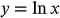.

Step-by-step explanation

Step 1.

Draw the graph, then left shit by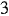units and then reflect about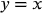Draw all the transformations of the given graph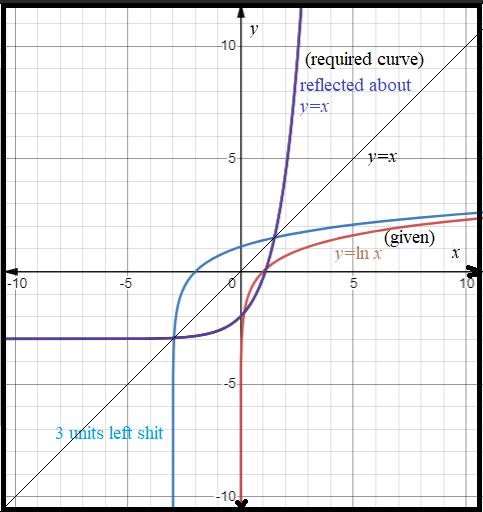From the above graph,

The equation of the required graph is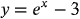.